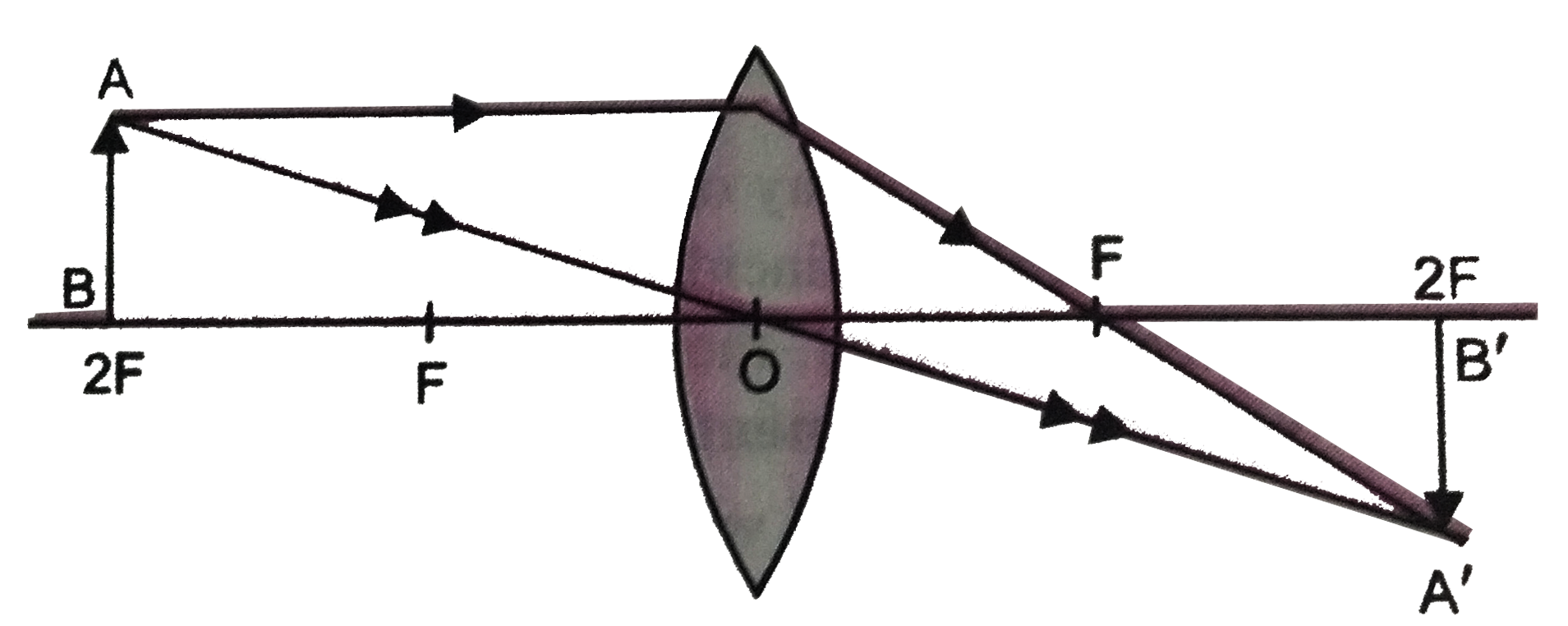# A convex lens is used to focus the rays from the far off light bulb at a distance of one meter from the lens. What should be the focal length of the l

110 views
in Physics
closed
A convex lens is used to focus the rays from the far off light bulb at a distance of one meter from the lens. What should be the focal length of the lens ? Draw a ray diagram to show the formation of image.

by (71.8k points)
selected by

The far off light bulb acts as an object at u = oo. Let its image be formed at a distance v from the lens.
From lens formula
(1)/(f) = (1)/(v) - 1/u = (1)/(v) - 1/oo = (1)/(v)
:. f =v =1 meter
Hence focal length of the lens = 1meter. The course of rays is as shown in fig.